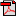# Lesson 4: Repeated Addition and Number Lines

## Activities

### Activity 1: Repeated Addition and Multiplication

Materials: fine point dry-erase markers (kit), whiteboard (kit)
Parent note: When relating multiplication to repeated addition, you can look at "4×2" as 4 groups of 2 or 2+2+2+2 OR as 4 being added twice or 4+4. Here, it's presented in the first way as this aligns with the idea of equal groups more closely. In the end, it doesn't really matter which way the sentence is interpreted because of the commutative property of multiplication (4×2=2×4), which is taught in an upcoming lesson.

Remind your child that multiplication is basically repeated addition or adding a number to itself more than once. Write 2+2+2+2 and 4×2 on the whiteboard, and say, "2+2+2+2 is asking you to add 2 four times, so it's the same as 4 groups of 2 or 4×2."

Now, write "2×6" on the whiteboard, and say, "This sentence has the factors 2 and 6. The first factor tells us how many times to add the second factor. So, this tells us to add 6 twice, or two times. Can you write the addition sentence?" She should write 6+6.
Repeat this process with the following multiplication sentences until it's clear that your child understands how to create repeated addition sentences out of multiplication sentences. Allow her to also find the products and sums using what she knows about addition and skip counting. For example, if she knows that 6+6 is equal to 12, she can use that to figure out 4×6 or 6+6+6+6.
• 4×6 (6+6+6+6)
• 7×3 (3+3+3+3+3+3+3)
• 5×1 (1+1+1+1+1)
• 1×8 (8)
• 3×10 (10+10+10)Student Activity Page